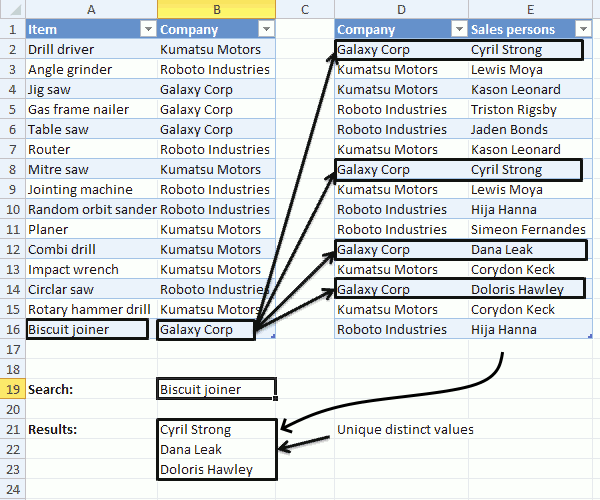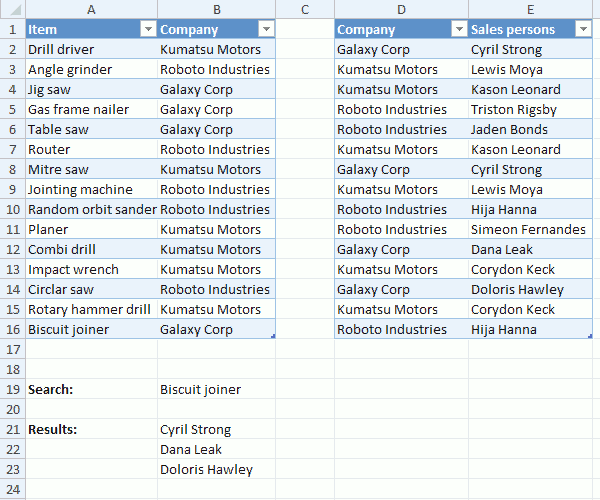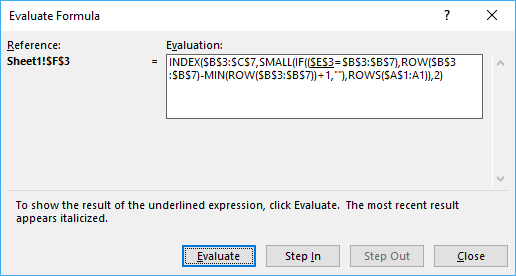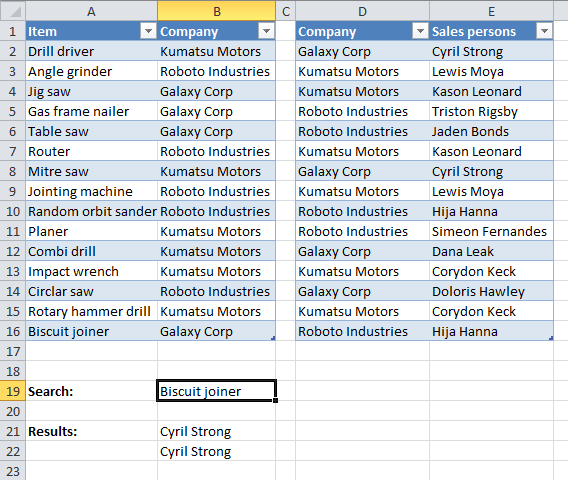Author: Oscar Cronquist Article last updated on October 17, 2019In this post, I am going to show you how to extract unique distinct values and duplicates using a formula, from a relational table. The image above shows a search value in cell B19, it is used by the formula to look for matches in A2:A16.

The formula finds a match in cell A16 and uses the corresponding value in cell B16 to do a second search in D2:D16, the two tables share values defined in B2:B16 and in D2:D16.

This makes it possible to perform searches across data sets, the value in cell B16 is found in cells D2, D8, D12 and D14. The corresponding cells in E2:E16 are E2, E8, E12 and E14, however, cell E2 and E8 contains duplicate values and the formula returns only one instance of those two values in cell range B21:B23.

In a previous post I described how to do lookups in a related table.

#### What is a relational table?

In a relational database (Microsoft Access), the data in one table is related to the data in other tables. In general, tables can be related in one of three different ways: one-to-one, one-to-many or many-to-many.  The relationship is used to cross-reference information between tables. Source: University of Sussex

### Unique distinct valuesArray formula in cell B21:

=IFERROR(INDEX(Table2[Sales persons], SMALL(IF((ISERROR(MATCH(Table2[Company], IF(\$B\$19=Table1[Item], Table1[Company], ""), 0)))+COUNTIF(\$B\$20:B20,Table2[Sales persons]), "", MATCH(ROW(Table2[Company]), ROW(Table2[Company]))), 1)), "")

To enter an array formula you copy above formula and paste to a cell. Press and hold CTRL and Shift simultaneously, then press Enter once. Release all keys.

The formula is now surrounded with curly brackets, don't enter these characters yourself, they appear automatically. {=formula}

Copy cell B21 and paste to cells below or simply select cell B21 and press and hold with left mouse button on the black dot located at the bottom right corner of the cell. Then drag with mouse down to cells below as far as needed, release left mouse button.

### Explaining formula in cell B21

The "Evaluate formula" feature lets you examine a formula calculation in more detail, simply select cell B21 and then go to tab "Formulas" on the ribbon.

Press with mouse on "Evaluate formula" button to open the Evaluate Formula dialog box, the "Evaluate" button located on the dialog box takes you through the calculation step by step which is great if you want to troubleshoot or simply understand a formula.(The image above does not show the actual formula used in this example.)

#### Step 1 - Find values equal to search value

The IF function has three arguments, IF(logical_test, [value_if_true], [value_if_false]). The first argument contains a logical expression, it returns an array containing values TRUE or FALSE.

The second argument will run if the logical expression returns TRUE and the third will run if the value is FALSE.

IF(\$B\$19=Table1[Item], Table1[Company], "")

becomes

IF("Biscuit joiner"=Table1[Item], Table1[Company], "")

becomes

IF("Biscuit joiner"={"Drill driver"; "Angle grinder"; "Jig saw"; "Gas frame nailer"; "Table saw"; "Router"; "Mitre saw"; "Jointing machine"; "Random orbit sander"; "Planer"; "Combi drill"; "Impact wrench"; "Circlar saw"; "Rotary hammer drill"; "Biscuit joiner"}, Table1[Company], "")

becomes

IF("Biscuit joiner"={"Drill driver"; "Angle grinder"; "Jig saw"; "Gas frame nailer"; "Table saw"; "Router"; "Mitre saw"; "Jointing machine"; "Random orbit sander"; "Planer"; "Combi drill"; "Impact wrench"; "Circlar saw"; "Rotary hammer drill"; "Biscuit joiner"}, {"Kumatsu Motors"; "Roboto Industries"; "Galaxy Corp"; "Galaxy Corp"; "Galaxy Corp"; "Roboto Industries"; "Kumatsu Motors"; "Roboto Industries"; "Roboto Industries"; "Kumatsu Motors"; "Kumatsu Motors"; "Kumatsu Motors"; "Roboto Industries"; "Kumatsu Motors"; "Galaxy Corp"}, "")

and returns

{"";"";"";"";"";"";"";"";"";"";"";"";"";"";"Galaxy Corp"}

#### Step 2 - Find relational values that corresponds to search value

The MATCH function returns the relative position of an item in an array or cell range that matches a specified value in a specific order.

MATCH(Table2[Company], IF(\$B\$19=Table1[Item], Table1[Company], ""), 0)

becomes

MATCH(Table2[Company], {"";"";"";"";"";"";"";"";"";"";"";"";"";"";"Galaxy Corp"}, 0)

becomes

MATCH({"Galaxy Corp"; "Kumatsu Motors"; "Kumatsu Motors"; "Roboto Industries"; "Roboto Industries"; "Kumatsu Motors"; "Galaxy Corp"; "Kumatsu Motors"; "Roboto Industries"; "Roboto Industries"; "Galaxy Corp"; "Kumatsu Motors"; "Galaxy Corp"; "Kumatsu Motors"; "Roboto Industries"}, {"";"";"";"";"";"";"";"";"";"";"";"";"";"";"Galaxy Corp"}, 0)

and returns

{15; #N/A; #N/A; #N/A; #N/A; #N/A; 15; #N/A; #N/A; #N/A; 15; #N/A; 15; #N/A; #N/A}

This array shows us where the matches are in the column. 15 is in first, seventh, eleventh and thirteenth position.

#### Step 3 - Identify errors

The formula errors out if we don't get rid of the error values, the ISERROR function returns TRUE if the value is an error and FALSE if not.

ISERROR(MATCH(Table2[Company], IF(\$B\$19=Table1[Item], Table1[Company], ""), 0))

becomes

ISERROR({15; #N/A; #N/A; #N/A; #N/A; #N/A; 15; #N/A; #N/A; #N/A; 15; #N/A; 15; #N/A; #N/A})

and returns

{FALSE; TRUE; TRUE; TRUE; TRUE; TRUE; FALSE; TRUE; TRUE; TRUE; FALSE; TRUE; FALSE; TRUE; TRUE}

#### step 4 - Avoid duplicate values

The COUNTIF function has two arguments COUNTIF(rangecriteria), the first argument has an expanding cell reference that grows when the cell is copied to cells below. This makes it aware of previously displayed values and we can now avoid duplicates.

COUNTIF(\$B\$20:B20,Table2[Sales persons])

becomes

COUNTIF("",Table2[Sales persons])

and returns

{0;0;0;0;0;0;0;0;0;0;0;0;0;0;0}

This array tells us that no values have been shown above cell B21.

#### Step 5 - Add arrays (OR logic)

By adding the two arrays we apply OR logic meaning that TRUE + TRUE = TRUE. FALSE + TRUE = TRUE. TRUE + FALSE = TRUE and FALSE + FALSE = FALSE.

Excel automatically converts boolean values to their numerical equivalents when the four basic arithmetic operations are preformed. Addition, subtraction, multiplication, and division.

(ISERROR(MATCH(Table2[Company], IF(\$B\$19=Table1[Item], Table1[Company], ""), 0)))+COUNTIF(\$B\$20:B20,Table2[Sales persons])

becomes

{FALSE; TRUE; TRUE; TRUE; TRUE; TRUE; FALSE; TRUE; TRUE; TRUE; FALSE; TRUE; FALSE; TRUE; TRUE} + {0;0;0;0;0;0;0;0;0;0;0;0;0;0;0}

and returns

{0;1;1;1;1;1;0;1;1;1;0;1;0;1;1}

#### Step 6 - Replace boolean values with row numbers and blanks

IF((ISERROR(MATCH(Table2[Company], IF(\$B\$19=Table1[Item], Table1[Company], ""), 0)))+COUNTIF(\$B\$20:B20,Table2[Sales persons]), "", MATCH(ROW(Table2[Company]), ROW(Table2[Company])))

becomes

IF({0;1;1;1;1;1;0;1;1;1;0;1;0;1;1}, "", MATCH(ROW(Table2[Company]), ROW(Table2[Company])))

becomes

IF({0;1;1;1;1;1;0;1;1;1;0;1;0;1;1}, "", {1; 2; 3; 4; 5; 6; 7; 8; 9; 10; 11; 12; 13; 14; 15})

and returns

{1;"";"";"";"";"";7;"";"";"";11;"";13;"";""}

#### Step 7 - Extract smallest row number

The SMALL function returns the smallest row number in the array.

SMALL(IF((ISERROR(MATCH(Table2[Company], IF(\$B\$19=Table1[Item], Table1[Company], ""), 0)))+COUNTIF(\$B\$20:B20,Table2[Sales persons]), "", MATCH(ROW(Table2[Company]), ROW(Table2[Company]))), 1)

becomes

SMALL({1;"";"";"";"";"";7;"";"";"";11;"";13;"";""}, 1)

and returns 1.

#### Step 8 - Return value from Table2[Sales persons]

The INDEx function returns a value from an array or cell range based on a row and column number.

INDEX(Table2[Sales persons], SMALL(IF((ISERROR(MATCH(Table2[Company], IF(\$B\$19=Table1[Item], Table1[Company], ""), 0)))+COUNTIF(\$B\$20:B20,Table2[Sales persons]), "", MATCH(ROW(Table2[Company]), ROW(Table2[Company]))), 1))

becomes

INDEX(Table2[Sales persons], 1)

becomes

INDEX({"Cyril Strong"; "Lewis Moya"; "Kason Leonard"; "Triston Rigsby"; "Jaden Bonds"; "Kason Leonard"; "Cyril Strong"; "Lewis Moya"; "Hija Hanna"; "Simeon Fernandes"; "Dana Leak"; "Corydon Keck"; "Doloris Hawley"; "Corydon Keck"; "Hija Hanna"}, 1)

and returns "Cyril Strong" in cell B21.

#### Step 9 - Remove error values

The IFERROR function removes errors that will show up when the formula runs out of values to display.

IFERROR(INDEX(Table2[Sales persons], SMALL(IF((ISERROR(MATCH(Table2[Company], IF(\$B\$19=Table1[Item], Table1[Company], ""), 0)))+COUNTIF(\$B\$20:B20,Table2[Sales persons]), "", MATCH(ROW(Table2[Company]), ROW(Table2[Company]))), 1)), "")

Note, be careful with the IFERROR function. It removes all kinds of errors which make it much harder for you to troubleshoot a formula.

### Duplicate valuesArray formula in cell B21:

=IFERROR(INDEX(Table6[Sales persons], SMALL(IF((NOT(ISERROR(MATCH(Table6[Company], IF(\$B\$19=Table5[Item], Table5[Company], ""), 0)))*COUNTIFS(Table6[Company],Table6[Company],Table6[Sales persons],Table6[Sales persons])>1), MATCH(ROW(Table6[Company]), ROW(Table6[Company])), ""), ROW(A1))), "")

How to create an array formula

1. Copy above array formula
2. Select cell B21
3. Press with left mouse button on in formula bar
4. Paste (Ctrl + v)
5. Press and hold Ctrl + Shift
6. Press Enter
7. Release all keys

The formula in the formula bar is now surrounded by curly brackets: {=array_formula}

How to copy an array formula

1. Select cell B21
2. Copy cell (Ctrl + c)
3. Select cell range B22:B23
4. Paste (Ctrl + v)

### Get the Excel fileUnique-distinct-values-related-tables2.xlsx